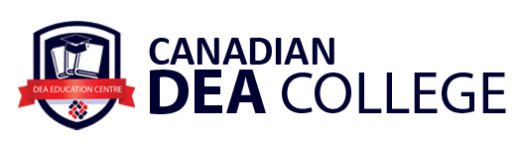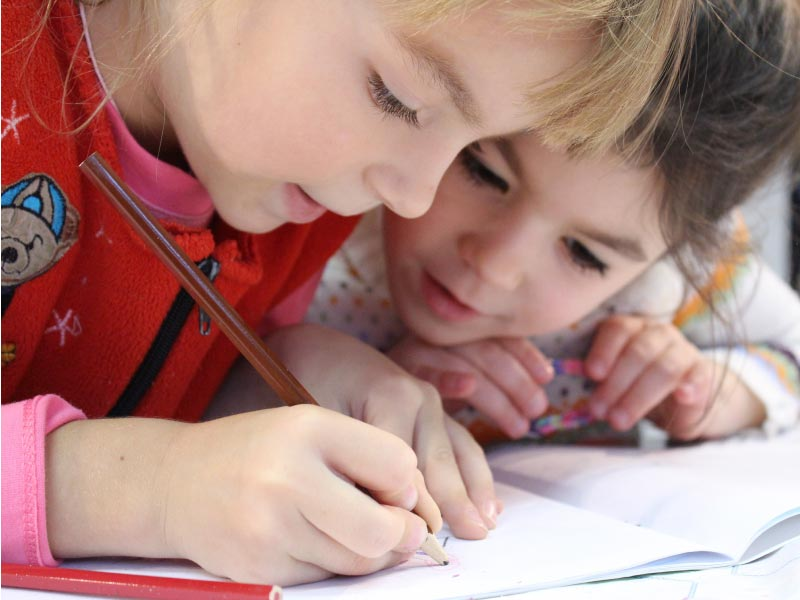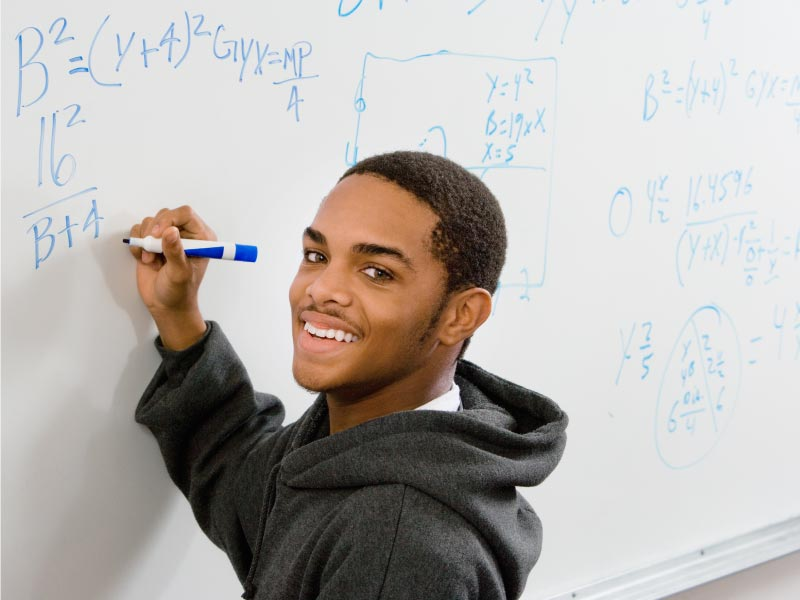# Online Mathematics Courses DEA College

Enrolled: 100 students
Duration: Per Hour### How we can help

We can help your child with understanding the basics, problem solving, pattern recognition, and solving equations. Our tutors are able to provide your child with help in various math subjects including core math, but also algebra, geometry, calculus and trigonometry.

Math is an essential skill in today’s world and working with numbers is an important part of life. At DEA we will work closely with your child to increase his or her skill set and to relate math problems to real life situation to make it fun and exciting to learn.

This program does not require approval by the registrar of the Private Training Institutions Branch (PTIB) of the Ministry of Advanced Education, Skills & Training.DEA math boot camp arouse the curiosity of students and encourage a deep personal involvement with the creative elements of mathematics and science due to the camps’ problem-solving sessions and contests.

Math camp gives learners a chance to broaden their mathematical horizons, particularly in those fields from where (for example) Olympiad problems are drawn. Rather than concentrating solely on problem solving, material covered familiarizes students with important mathematical concepts and widely applicable methods of proof. These camps help inspire learning and a love of mathematics that lasts into college and beyond, helping students significantly improve their performance and increase their understanding.

• grade 3· Number sequence to 10
· Familiar number arrangements up to objects
· One-to-one correspondence
· Numbers in-depth to 10
· Repeating patterns of two of three elements
· Direct comparison for length, mass & volume
· Single attribute of 3-D objects
• · Skip counting starting at 0 to 100
· Arrangements up to 10 objects
· Numbers in-depth to 20
· Addition & subtraction to 20
· Mental math strategies to 18
· Repeating patterns of two to four elements
· Representation of pattern
· Equalities & inequalities
· Symbol for equality
· Process of measurement using comparison
· One attribute of 3-D objects & 2-D shapes
· Composite 2-D shapes & 3-D objects
· 2-D shapes in the environment
• · Skip counting at starting points other than 0 to 100
· Numbers in-depth to 100
· Even, odd & ordinal numbers
· Addition & subtraction to 100
· Mental math strategies to 18
· Repeating patterns of three to five elements
· Increasing patterns
· Equality & inequality
· Symbols for equality & inequality
· Days, weeks, months, & years
· Non-standard units of measure for length, height distance around, mass (weight)
· Two attributes of 3-D objects and 2-D shapes
· Cubes, spheres, cones, cylinders, pyramids
· Triangles, squares, rectangles, circles
· 2-D shapes in the environment
· Data about self and others
· Concrete graphs and pictographs
• · Skip counting at starting points other than 0 to 1000
· Numbers in-depth to 1000
· Addition & subtraction to 1000
· Mental math strategies for 2-digit numerals
· Multiplication up to 5 x 5
· Representation of fractions
· Increasing patterns
· Decreasing patterns
· One-step addition and subtraction equations
· Non-standard & standard units of time
· Measurements of length (cm, m) & mass (g, kg)
· Perimeter of regular & irregular shapes
· Faces, edges & vertices of 3-D objects
· Triangles, quadrilaterals, pentagons, hexagons, octagons
· First-hand data
· Bar graphs
• · Numbers in-depth to 10 000
· Addition & subtraction to 10 000
· Multiplication & division of numbers
· Fractions less than or equal to one
· Decimals to hundredths
· Patterns in tables & charts
· Symbols to represent unknowns
· One-step equations
· Digital clocks, analog clocks, & calendar dates
· Area of regular & irregular 2-D shapes
· Rectangular & triangular prisms
· Line symmetry
· Many-to-one correspondence including bar graphs & pictographs

· Numbers in-depth to 1 000 000
· Estimation strategies for calculations & problem solving
· Mental mathematics strategies for multiplication facts to 81 & corresponding division facts
· Mental mathematics for multiplication
· Multiplication for 2-digit by 2-digit & division for 3-digit by 1-digit
· Decimal & faction comparison
· Addition & subtraction of decimals
· Prediction using a pattern rule
· Single-variable, one-step equations with whole number coefficients & solutions
· Perimeter & area of rectangles
· Length, volume, & capacity
· Parallel, intersecting, perpendicular, vertical & horizontal edged & faces
· Rectangles, squares, trapezoids, parallelograms & rhombuses
· 2-D shape single transformation
· First—hand & second-hand data
· Double bar graphs
· Likelihood of a single outcome

· Numbers in-depth greater than 1 000 000 & smaller than one thousandth
· Factors & Multiples
· Improper fractions & mixed numbers
· Ratio & whole number percent
· Integers
· Multiplication & division of decimals
· Order of operations excluding exponents
· Patterns & relationships in graphs & tables including tables of value
· Letter variable representation of number relationships
· Preservation of equality
· Perimeter & area of rectangles
· Length, volume, & capacity
· Types of triangles
· Regular & irregular polygons
· Combinations of transformations
· Single transformation in the first quadrant of the Cartesian plane
· Line graphs
· Methods of data collection
· Graph data
· Experimental & theoretical probability

· Divisibility rules
· Addition, subtraction, multiplication, & division of numbers
· Percents from 1% to 100%
· Decimal & fraction relationships for repeating & terminating decimals
· Addition & subtraction of positive fractions & mixed numbers
· Addition & subtraction of integers
· Table of values & graphs of linear relations
· Preservation of equality
· Expressions & equations
· One-step linear equations
· Properties of circles
· Area of triangles, parallelograms, & circles
· Geometric constructions
· Four quadrants of the Cartesian plane
· Transformations in the four quadrants of the Cartesian plane
· Central tendency, outliers & range
· Circle graphs
· Ratios, fractions, & percents to express probabilities
· Two independent events
· Three diagrams for two independent events

· Logic and patterns to solve games and puzzles
· Percents less than 1 and greater than 100 (decimal and fractional percents)
· Percent squares and cubes
· Square roots and Pythagorean Theorem
· Rates and proportional reasoning, ratio, proportions, and percent
· Operations with fractions (addition, subtraction, multiplication, division, and order of operations)
· Expressions and equations, writing and evaluating using substitution
· Two-step equations with integer coefficients, constants, and solutions
· Numerical proportional reasoning
· Surface area and volume of regular solids (right prisms, triangular prism, and cylinder)
· Construction, views, and nets of 3D objects
· Theoretical probability with two independent events
· Financial literacy – best buys (e.g., coupons, proportions, unit price, products, and services)

· Numerical and spatial reasoning, logic, and patterns to solve puzzles and games
· Operations with polynomials, of degree less than or equal to two
· Types of income (e.g., wages, salary, piece work, commission)
· Operations with rational numbers (addition, subtraction, multiplication, division, and order of operations)
· Rational numbers and order of operations
· Two-variable linear relations, using graphing, interpolation, and extrapolation
· Multi-step one-variable linear equations, including distribution and rational coefficients, constants, and solutions
· Spatial proportional reasoning (e.g., scale diagrams, similar triangles, linear unit conversions)
· Probability and statistics in society (e.g. sampling techniques, misleading stats)
· Financial literacy – Simple budgets and transactions (e.g., banking, interest, savings, planned purchases)

• Students are expected to know the following:
· operations on powers with integral exponents
· prime factorization
· functions and relations: connecting data, graphs, and situations
· linear functions: slope and equations of lines
· arithmetic sequences
· systems of linear equations
· multiplication of polynomial expressions
· polynomial factoring
· primary trigonometric ratios
· financial literacy: gross and net pay

· Strategies to solve puzzles and win games
· Primary trigonometric ratios
· Metric and imperial measurement conversions
· Analysis of measures of central tendency, including outliers
· Experimental probability
· Income, including taxes and deductions
· Savings and compound interest
· Area and volume
· Relations among data, graphs, and situations
· Powers with integral exponents
· Multiplication of polynomial expressions
· Common factors and trinomial factoring
· Linear equations with rational coefficients
· Linear relations

• Students are expected to know the following:
· create, interpret, and critique graphs
· primary trigonometric ratios
· metric and imperial measurement and conversions
· surface area and volume
· central tendency
· experimental probability
· financial literacy: gross and net pay

· Geometric/spatial reasoning to solve puzzles and games
· Primary trigonometric ratios
· Metric and imperial measurement conversions
· How to analyze measures of central tendency, including outliers
· Experimental probability
· Income
· Savings and compound interest
· How to calculate area and volume
· Relations among data, graphs, and situations
· Angles
· Tessellations

• Students are expected to know the following:
· real number system
· powers with rational exponents
· polynomial factoring
· rational expressions and equations
· trigonometry: non-right triangles and angles in standard position
· financial literacy: compound interest, investments, loans

· Strategies to solve puzzles and win games
· Deductive and inductive mathematical reasoning
· Trigonometry
· How probability and statistics are used in the real world
· Finances
· Arithmetic sequences/series
· How to factor polynomial expressions of the form:
· Rational expressions/equations
· Exponent laws
· Irrational numbers
· How to solve linear systems
· Quadratic functions/equations, including function notation

• Students are expected to know the following:
· forms of mathematical reasoning
· angle relationships
· graphical analysis:
— linear inequalities
— systems of equations
— optimization
· applications of statistics
· scale models
· financial literacy: compound interest, investments, and loans

· Strategies to solve puzzles and win games
· Deductive and inductive mathematical reasoning
· Angles and triangles
· Trigonometry
· How probability and statistics are used in the real world
· Finances
· Systems of linear inequalities
· How to conduct a math research project

• Students are expected to know the following:
· financial literacy: personal investments, loans, and budgeting
· rate of change
· how probability and statistics are used in different contexts
· interpreting graphs in society
· 3D objects: angles, views, and scale diagrams

· Strategies to solve puzzles and win games
· Deductive and inductive mathematical reasoning
· Trigonometry
· How probability and statistics are used in the real world
· Finances
· Scale diagrams
· 3D objects
· Slope as a rate of change
· Unit analysis

· Strategies to solve puzzles and win games
· Trigonometry
· How to factor polynomials of the form: including difference and sum of cubes
· Polynomial functions, including inequalities
· Geometric sequences, series
· Logarithms
· Exponential and logarithmic functions/equations
· Transformations of functions
· Compositions of functions
· Conics

Students are expected to know the following:
· geometric explorations:
— constructions
— conics
— fractals
· graphical representations of polynomial, logarithmic, exponential, and sinusoidal functions
· regression analysis
· combinatorics
· odds, probability, and expected value
· financial planning

· Strategies to solve puzzles and win games
· Scale diagrams and scale factors
· 3D drawings and spatial sense
· Set theory and conditional statements
· Odds and probability
· Combinatorics
· Regressions
· How to conduct a mathematics research project

Students are expected to know the following:
· measuring: using tools with graduated scales; conversions
using metric and imperial
· similar triangles: including right-angle trigonometry
· 2D and 3D shapes: including area, surface area, volume,
and nets
· 3D objects and their views (isometric drawing, orthographic projection)
· mathematics in the workplace
· financial literacy: business investments and loans

· Strategies to solve puzzles and win games
· Scale diagrams, scale factors
· Applications of trigonometry
· 3D drawings and spatial sense
· How to use measuring tools with precision and accuracy
· Transformations on 2D and 3D shapes
· Linear relationships

Students are expected to know the following:
· geometric constructions
· parallel and perpendicular lines:

— circles as tools in constructions
— perpendicular bisector
· circle geometry
· constructing tangents
· transformations of 2D shapes:
— isometries
— non-isometric transformations
— non-Euclidean geometries

· Strategies to solve puzzles and win games
· Circle geometry
· Constructions
· Apollonius’ problems
· Affine transformations of 2D shapes, including shearing
· Conic sections
· Coordinate geometry
· Non-Euclidean geometries

Students are expected to know the following:
· functions and graphs
· limits:
— left and right limits
— limits to infinity
— continuity

· differentiation:
— rate of change
— differentiation rules
— higher order, implicit
— applications
· integration:
— approximations
— the fundamental theorem of calculus
— methods of integration
— applications

· Strategies to solve puzzles and win games
· History of calculus
· Limits
· Derivatives
· Applications of derivatives
· Anti-differentiation
· Integration
· Fundamental theorem of calculus

### Who We Are

DEA goal is to provide students with a welcoming environment that is student-centered and flexible to accommodate each student’s interests and needs. We believe learning through role-plays, field trips and group learning activities help students integrate and advance more quickly. DEA creates an atmosphere that encourages all students equally in self-expression. We build students vocabulary for formal and informal situations by increasing communicative skills through appropriate question and answer techniques and presentations by eliciting students to self-correct.

This program does not require approval by the registrar of the Private Training Institutions Branch (PTIB) of the Ministry of Advanced Education, Skills & Training.